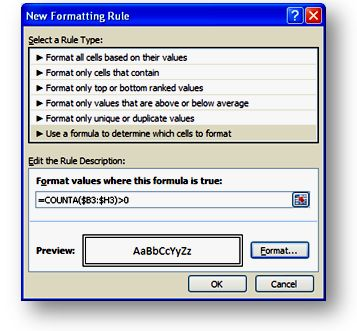# Automatically Creating borders if a cell has contents (Excel Conditional Format Training)

Gridlines Added Automatically As Data Is Entered

Wouldn’t it be cool if you could have gridlines (i.e. cell borders) magically appear on your worksheet only when you enter something in a row? You can accomplish this with Conditional Formatting (CF).Here’s how…

1) Select an area that you want gridlines to appear only on rows where something is entered (click the top left cell and drag to bottom right. e.g. B3:H15);

Use Conditional Formatting to automatically add gridlines

2) From the Home tab, click Conditional Formatting in the Styles group;

3) Click New Rule…;

4) In the ‘Select a Rule Type’ area select ‘Use a formula to determine which cells to format’;

5) In the ‘Format values where this formula is true’ field enter this formula..

=COUNTA(\$B3:\$H3)>0

…where B3 is the top left cell of your selected range and H3 is the top right cell of your selected range.

Use Conditional Formatting to automatically add gridlines
To choose formatting, click the Format… button, click the Border tab and select the Outline border and click OK.

Explanation of the CF formula:

COUNTA is a function that counts the number of values (text or numeric) in the range B3:H3. If that value is greater than zero (i.e. >0), the formatting is applied to all cells on that row.
The Absolute column references (\$) force the conditional formatting to be applied to all cells between column B and H.
Since we want to apply the formatting to a row only if there is something entered anywhere in that particular row, we use relative (no \$ signs) row referencing in the formula.
Since the range of cells that we selected to apply Conditional Formatting to contains more than one row, the Conditional Formatting formula will adjust relative to the other rows (although you won’t see those formulas in the CF dialog). Therefore, the condition for row  4 will be =COUNTA(\$B4:\$H4)>0 and row 5 will be =COUNTA(\$B5:\$H5)>0.

6) Now, when you type something in any cell in row 3, gridlines will be added from C4 to H4.

Use Conditional Formatting to automatically add gridlines

You will notice also that if you skip a row and enter something on the next row, there will be a gap in the gridlines from top to bottom.

Use Conditional Formatting to automatically add gridlines

To avoid this we need to make an adjustment to our CF formula.

7) Select the same range you selected in Step 1 and click Home (tab), Conditional Formatting, Manage Rules…;

8) Click Edit Rule… and in the ‘Format values where this formula is true’ field, replace the previous formula with this one and click OK.

=OR(COUNTA(\$B3:\$H3)>0,COUNTA(\$B4:\$H\$15)>0)

Explanation of this formula:

The second part of this rule COUNTA(\$B4:\$H\$15) counts if there is anything entered in any rows below the relative cell. If there is anything in the rows below, the COUNTA result will be greater than (>) 0 and the gridline formatting will be applied to the relative row.
The OR function allows us to use both formulas (COUNTA(\$B3:\$H3)>0 and COUNTA(\$B4:\$H\$15)>0) to check for two conditions. If the first condition is true OR if the second condition is true, the result of the entire formula will be TRUE and Conditional Formatting will be applied to the entire row.
The row numbers in the formulas are critical for this to work. The first formula COUNTA(\$B3:\$H3)>0 must contain the row number (e.g. 3) of the the Active Cell (e.g. B3) of the range you have selected. The first reference in the second formula (e.g. \$B4) must contain the number of the row immediately below the Active Cell. The second reference must contain the number of the last row in the range (e.g. \$H\$15) and we can make the row reference Absolute by preceding it with  a dollar sign (\$15).
Now, with this new formula, if there are empty rows between values, the empty rows will have borders applied because the count of values below is >0 and therefore the condition is TRUE and the formatting is applied.

Note that this particular formatting will not work if there are formulas in the Conditional Format range since the formulas will be counted. Therefore, formatting would be applied to all rows with formulas. To make this work with formulas, you would need separate CF rules for the cells containing formulas. If you try this and get it to work, send me a sample worksheet.

Conditional Formatting is so versatile that you can create automatic formatting for almost any condition. It just takes some practice to understand how it works. The key lies in the application of Relative and Absolute referencing in relation to the Active Cell of the selected range.

## 3 thoughts on “Automatically Creating borders if a cell has contents (Excel Conditional Format Training)”

1. Hi,

Can you please tell me how to separate CF rules for the cells containing formulas in excel 2007. Because in my work sheet I don’t want to create a border for empty cells which contains formulas.

Thanks
Sandun

• I’m not sure what you mean about separating conditional format rules. Can you give a more specific examples and I’ll do my best to help.

2. I use the following to format rows that contain formulas dynamically. If the formula doesn’t return anything (no data on the row/range) the cells are not formatted. If any cell formula on that row/range returns data the cells are formatted.

This works because the test =LEN() will return 0 even if there is a formula in the cell, whereas =ISBLANK returns FALSE whether the formula is true or not.

=NOT(LEN(\$A5:\$W5)=0)